To find a loop or cycle and remove it in a linked list we have two approaches.
1. Using the visited flag in each node.
2. Hare and Tortoise/ Floyd's cycle detection algorithm

## Using the visited flag method to find a loop in a linked list

using this method we set a flag to value true for each node that visited the first time. and if we visit the node again that has a flag set to value true then the list has a loop and if not then the list has not any cycle or loop.
but using this method we need to update the whole list so that is why we don't use this method to find a loop in the list.

instead, we use the hare and tortoise algorithm to find the cycle in the list.

## Hare and Tortoise algorithm to find a loop in the linked list.

this algorithm is also called Floyd's cycle detection algorithm. in this algorithm, we use two reference tortoises and a hare. in the beginning, both reference hare and tortoise are set to the first node of the linked list.

in every iteration, the hare reference visits two nodes at a time and the tortoise reference visits a single node at a time. and if both references meet on the same node then the list has a loop and if not then the list does not have any loop.

#### let's understand the algorithm with an example

let's say we have a linked list that has 8 nodes. as you see in the image given below. and it has a cycle in it.

so first we set two references hare and tortoise and at the beginning of the iteration, we set both references to the first node of the list.

as we know the tortoise reference visits one node at a time and the hare reference visits two nodes at a time.so now both the reference meets at the same node then the list has a cycle.

```def has_cycle(self):
if self.find_cycle() is None:
return False    else:
return True
def find_cycle(self):
if self.start is None or self.start.link is None:
return None
slowR = self.start
fastR = self.start

while fastR is not None and fastR.link is not None:
if slowR == fastR:
return slowR
return None```

To remove a loop in the linked list first we need to find the length of the loop in the linked list and then we need to find the length of the rest of the linked list and then we the length of the loop and length of the rest of the linked list. so we get the whole length of the list and then we place the null or None at the last node of the list.

## Remove loop in a linked list

after we can find the reference p and q.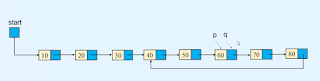First, we need to find the length of a loop in the linked list.

### Length of the loop in the linked list

so to find the length of the loop in the linked list we traverse one reference one node at a time among both references and we count the number of nodes that traverse by the reference.

and when the one reference value becomes equal to the second reference then we stop traversing. and the number of nodes that a reference traverse is the length of the loop in the linked list.

as you see we move the q reference one node at a time and count the number of nodes that the q variable traverse before the value of node q becomes equal to the node p.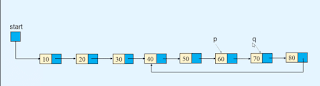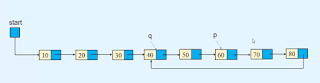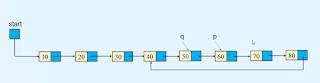so after traversing the 5 nodes the value of node q become equal to the value of the node p. so the length of the loop in the linked list is 5.

now we need to find the length of the rest of the linked list.

### Lenght of rest of the linked list

to find the length of the rest of the linked list first we place a reference to the first node of the list among both of the references. as you see in the image given below we place the reference to the first node of the list.now we traverse one node at a time using both of the references in the list until both references meet at the same node and count the number of nodes traverse by anyone reference. as you see in the image given below.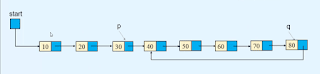as you see after traversing the three nodes both references meet at the same node. so now the number of nodes that traverse by anyone reference has become the remaining length of the list.

so now the length of the whole list is equal to the length of the cycle plus length of the remaining list.
in the above example, the total length of the list is equal to 5+3=8.

now we place the Null or None value in the linked part of the 8th node. as you see in the image given below.so now we remove the loop from the list.

```def remove_cycle(self):
c = self.find_cycle()
if c is None:
return    print("Node at which the cycle was detected is ", c.info)

p = c
q = c
len_cycle = 0
while True:
len_cycle+=1        q = q.link
if p == q:
break    print("Length of cycle is :", len_cycle)

len_rem_list = 0    p = self.start
while p != q:
len_rem_list+=1        p = p.link

print("Number of nodes not included in the cycle are : ", len_rem_list)
length_list = len_cycle + len_rem_list
print("Length of the list is : ", length_list)

p = self.start
for i in range(length_list-1):

#### Python program for traversing in the linked list.

```class Node:

def __init__(self, value):
self.info = value

def __init__(self):
self.start = None
def display_list(self):
if self.start is None:
print("List is empty")
return        else:
print("List is :   ")
p = self.start
while p is not None:
print(p.info, " ", end=' ')
print()

def count_nodes(self):
p = self.start
n = 0        while p is not None:
n += 1            p = p.link
print("Number of nodes in the list = ", n)

def search(self):
position = 1        p = self.start
while p is not None:
if p.info == x:
print(x, " is at position ", position)
return True            position += 1            p = p.link
else:
return False
def insert_in_beginning(self, data):
temp = Node(data)
self.start = temp

def insert_at_end(self, data):
temp = Node(data)
if self.start is None:
self.start = temp
return
p = self.start
while p.link is not None:

def create_list(self):
n = int(input("Enter the number of nodes  :  "))
if n == 0:
return        for i in range(n):
data = int(input("Enter the element to be inserted : "))
self.insert_at_end(data)

def insert_after(self, data, x):
p = self.start
while p is not None:
if p.info == x:
break            p = p.link

if p is None:
print(x, "not present in the list")
else:
temp = Node(data)

def insert_before(self, data, x):
# If list is empty        if self.start is None:
print("List is empty")
return
if x == self.start.info:
temp = Node(data)
self.start = temp
return

p = self.start
while p.link is not None:
if p.link.info == x:
break            p = p.link

if p.link is None:
print(x, " not present in the list")
else:
temp = Node(data)

def insert_at_position(self, data, k):
if k == 1:
temp = Node(data)
self.start = temp
return
p = self.start
i = 1        while i < k - 1 and p is not None:
i += 1
if p is None:
print("You can insert only upto position", i)
else:
temp = Node(data)

def delete_node(self, x):

if self.start is None:
print("List is empty")

if self.start.info == x:
return
p = self.start
while p.link is not None:
if p.link.info == x:
break            p = p.link

if p.link is None:
print("Element ", x , "not in list")
else:

def delete_first_node(self):
if self.start is None:
return        self.start = self.start.link

def delete_last_node(self):

if self.start is None:
return
if self.start.link is None:
self.start = None            return
p = self.start
def reverse_list(self):

prev = None        p = self.start
while p is not None:
prev = p
p = next
self.start = prev

def bubble_sort_exdata(self):

end = None
while end != self.start.link:
p = self.start
while p.link != end:
if p.info > q.info:
p.info, q.info = q.info, p.info
end = p

end = None
while end != self.start.link:
r = p = self.start
while p.link != end:
if p.info > q.info :
if p != self.start:
else:
self.start = q
p,q = q,p
r = p
end = p

def has_cycle(self):
if self.find_cycle() is None:
return False        else:
return True
def find_cycle(self):
if self.start is None or self.start.link is None:
return None
slowR = self.start
fastR = self.start

while fastR is not None and fastR.link is not None:
if slowR == fastR:
return slowR
return None
def remove_cycle(self):
c = self.find_cycle()
if c is None:
return        print("Node at which the cycle was detected is ", c.info)

p = c
q = c
len_cycle = 0
while True:
len_cycle+=1            q = q.link
if p == q:
break        print("Length of cycle is :", len_cycle)

len_rem_list = 0        p = self.start
while p != q:
len_rem_list+=1            p = p.link

print("Number of nodes not included in the cycle are : ", len_rem_list)
length_list = len_cycle + len_rem_list
print("Length of the list is : ", length_list)

p = self.start
for i in range(length_list-1):

def insert_cycle(self, x):
if self.start is None:
return        p = self.start
px = None        prev = None
while p is not None:
if p.info == x:
px = p
prev = p

if px is not None:
else:
print(x, " not present in list")

def merge2(self, list2):
merge_list.start = self._merge2(self.start, list2.start)
return merge_list

def _merge2(self, p1, p2):
if p1.info <= p2.info:
startM = p1
else:
startM = p2
pM = startM

while p1 is not None and p2 is not None:
if p1.info <= p2.info:
else:

if p1 is None:
else:

return startM

def merge_sort(self):
self.start = self._merge_sort_rec(self.start)

def _merge_sort_rec(self, list_start):

if list_start is None or list_start.link is None:
return list_start

start1 = list_start
start2 = self._divide_list(list_start)
start1 = self._merge_sort_rec(start1)
start2 = self._merge_sort_rec(start2)
startM = self._merge2(start1, start2)

return startM

def _divide_list(self, p):
while q is not None and q.link is not None:
p.link = None        return start2

##########################

list.create_list()

while True:
print("1. Display list")
print("2. Count the number of nodes")
print("3. Search for an element")
print("4. Insert in empty list/insert in beginning of the list")
print("5. Insert a node at the end of the list")
print("6. Insert a node after a specified node")
print("7. Insert a node before a specified node")
print("8. Insert a node at a given position")
print("9. Delete first node")
print("10. Delete last node")
print("11. Delete any node")
print("12. Reverse the list")
print("13. Bubble sort by exchanging data")
print("14. Bubble sort by exchanging links")
print("15. Merge sort")
print("16. Insert Cycle")
print("17. Detect Cycle")
print("18. Remove Cycle")
print("19. Quite")

option = int(input("Enter your choice"))

if option == 1:
list.display_list()
elif option == 2:
list.count_nodes()
elif option == 3:
data = int(input("Enter the element to be searched : "))
list.search(data)
elif option == 4:
data = int(input("Enter the element to be inserted : "))
list.insert_in_beginning(data)
elif option == 5:
data = int(input("Enter the element to be inserted : "))
list.insert_at_end(data)
elif option == 6:
data = int(input("Enter the element to be inserted : "))
x = int(input("Enter the element after which to insert : "))
list.insert_after(data, x)
elif option == 7:
data = int(input("Enter the element to be inserted : "))
x = int(input("Enter the element before which to insert : "))
list.insert_before(data, x)
elif option == 8:
data = int(input("Enter the element to be inserted : "))
k = int(input("Enter the position at which to insert : "))
list.insert_at_position(data, k)
elif option == 9:
list.delete_first_node()
elif option == 10:
list.delete_last_node()
elif option == 11:
data = int(input("Enter the element to be deleted : "))
list.delete_node(data)
elif option == 12:
list.reverse_list()
elif option == 13:
list.bubble_sort_exdata()
elif option == 14:
elif option == 15:
list.merge_sort()
elif option == 16:
data = int(input("Enter the element at which the cycle has to be inserted : "))
list.insert_cycle(data)
elif option == 17:
if list.has_cycle():
print("List has a cycle")
else:
print("List does not have a cycle")
elif option == 18:
list.remove_cycle()
elif option == 19:
break    else:
print("Wrong option")
print()```